# A.C. Induction Motor Design and Operating Principles

## Induction Motor Design Principle

We all know that an electric motor is used for the conversion of electrical energy into mechanical energy. This mechanical energy may be used for the pumping of liquid from one place to other by using pumps or even to blow air by blowers or ceiling fans. The conversion of electrical power to mechanical energy takes place in the rotating part of the motor. In D.C. Motors, the electric power is conducted directly to the armature (the rotating part) through brushes & commutator. Thus we can say a D.C. Motor as a conduction motor. But in case of an A.C. Motor, the rotor does not receive electric power by conduction, but by Induction. Thus they are called as induction motors. This can be compared with the secondary winding of a transformer. These induction motors are also called as rotating transformers. Of all motors, it is generally a 3-phase or a poly-phase induction motor is used in a larger extent in many industries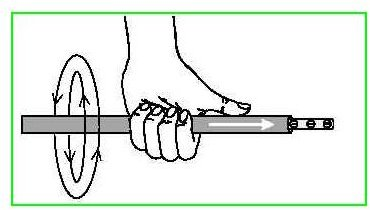The Direction of rotation of an Electric motor is given by Fleming’s Left Hand rule:

• It shows the relation between the direction of "thrust" on a conductor carrying a "current" in a "magnetic field".
• Keep the Thumb, Index finger & the Middle finger of the left hand at right angles to each other.The First finger or the index finger indicates the direction of the Field.
• The seCond finger or the middle finger represents the direction of the Current.
• The THumb represents the direction of the THrust or the direction of motion of the conductor.

Also other important Law is the Faraday’s Law Of Electro Magnetic Induction.There are 3 important rules/laws of electro magnetic induction.They are as follows:

1. An EMF is induced in a coil whenever the flux through the coil changes with time.

2. The magnitude of induced EMF is directly proportional to the rate of change of flux.

3. The direction of the EMF is such as to oppose the change in flux.

These basic Laws govern the working of an electric motor. Remembering all the above laws, let us find out how the rotor rotates..!

## Why Does A Rotor Rotate?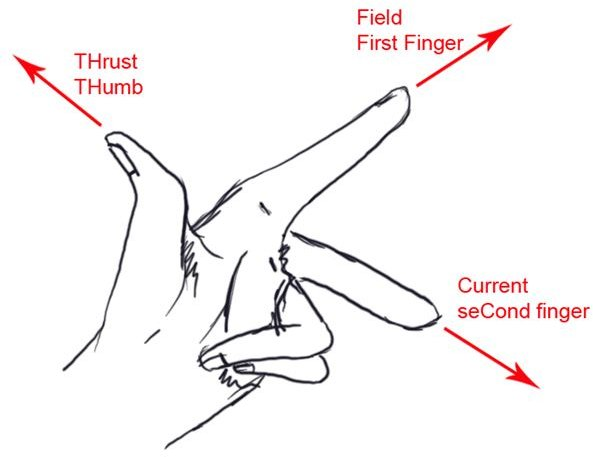If a 3-phase supply is fed to the stator windings of a 3-phase motor, a magnetic flux of constant magnitude, rotating at synchronous speed is set up. At this point, the rotor is stationary. The rotating magnetic flux passes through the air gap between the stator & rotor and sweeps past the stationary rotor conductors. This rotating flux, as it sweeps, cuts the rotor conductors, thus causing an e.m.f to be induced in the rotor conductors. As per the Faraday’s law of electromagnetic induction, it is this relative motion between the rotating magnetic flux and the stationary rotor conductors, which induces an e.m.f on the rotor conductors. Since the rotor conductors are shorted and form a closed circuit, the induced e.m.f produces a rotor current whose direction is given by Lenz’s Law, is such as to oppose the cause producing it. In this case, the cause which produces the rotor current is the relative motion between the rotating magnetic flux and the stationary rotor conductors. Thus to reduce the relative speed, the rotor starts to rotate in the same direction as that of the rotating flux on the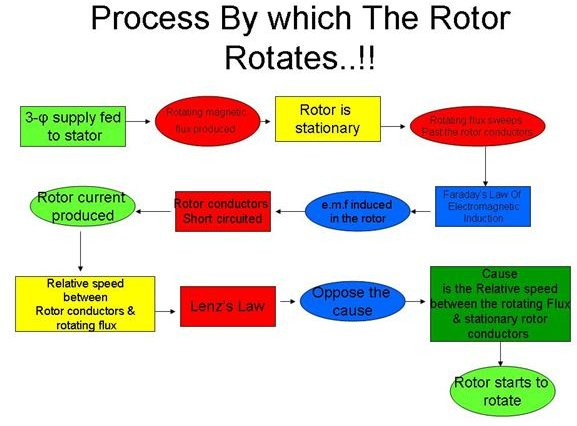stator windings, trying to catch it up. The frequency of the induced e.m.f is same as the supply frequency.

## Cogging Of Induction Motors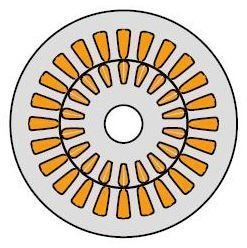Sometimes, when the supply voltage is low, the squirrel cage induction motor refuses to start. This happens when the number of stator teeth and the number of the rotor teeth is equal, thus causing a magnetic locking between the stator and the rotor. This phenomenon is other-wise called as teeth-locking or Magnetic locking. This problem can be overcome by having the number of rotor slots prime to the stator slots.

## Plugging of Motor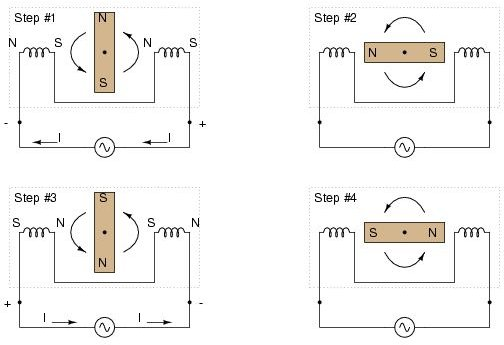The Induction motor can be stopped immediately by just interchanging any two of the stator leads. When an induction motor is rotating at a high speed, during emergency if situation arises that the motor has to be stopped immediately, can be done by interchanging any 2 leads of the stator supply. By doing this, it reverses the direction of the revolving flux, which produces a torque in the reverse direction, thus causing a breaking effect on the rotor. This breaking period is called the “Plugging” period.

## Analogy with the Mechanical ClutchThe Rotor Cu loss = Slip * Rotor Input

This is evident by considering the working of mechanical clutch, used in automobiles. By visualizing the figure pf plate clutch, it is evident that the torque on the drive shaft must be equal to the torque on the driven shaft. Further, it should be emphasized that the these two torques are the one and the same, because the torque is caused by the friction between the two plates. Let \$1 and \$2 be the angular velocities on these two shafts assuming the plate clutch is slipping.

Then input = T*ω1

Output= T* ω 2

But ω 2 = ω 1*(1-s)

So output = T* ω 1*(1-s)

Loss = T* ω 1 – T* ω 2

Loss = T* ω 1 – T ω 1*(1-s)

Loss = s * T ω 1

Thus

Loss = slip * input.

## Advantages & Disadvantages

Advantages of A.C. Induction Motors:

1. It has a simple design, low initial cost, rugged construction almost unbreakable

2. The operation is very simple with almost very less maintenance as there are no brushes.

3. The efficiency of these motors is very high, as there are no frictional losses, with reasonably good power factor.

4. The control gear for the starting purpose of these motors is minimum and thus simple and reliable operation.

Disadvantages of A.C. Induction Motors:

1. The speed control of these motors is not easy without some loss in efficiency.

2. As the load on the motor increases, the speed decreases.

3. The starting torque is inferior when compared to D.C. Motors.

## Motor Losses & Efficiency: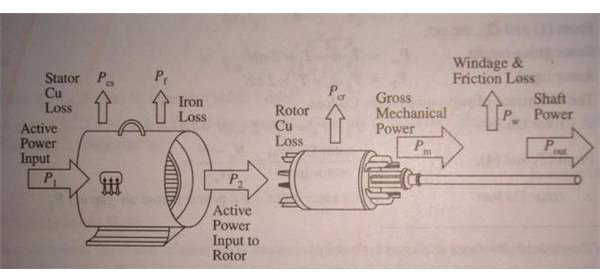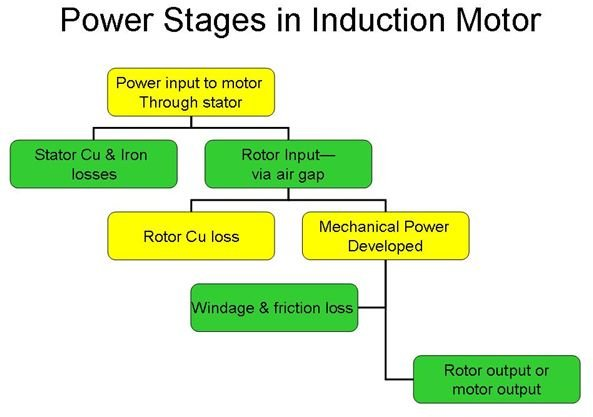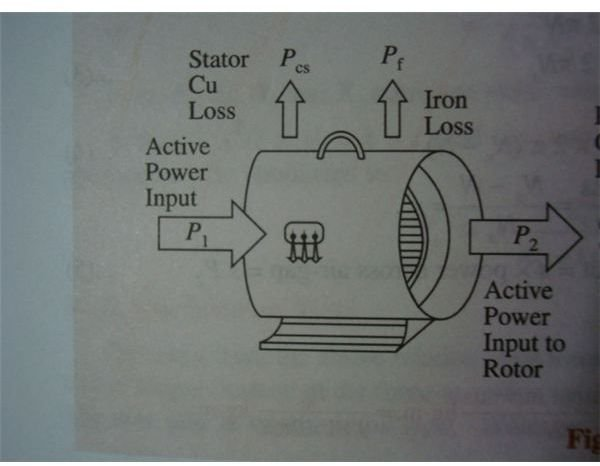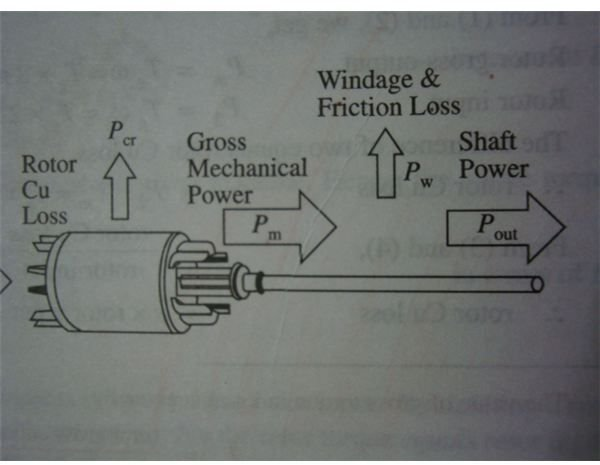## Image Credits

energymanagertraining.com

geocities.com

microchip.com

allaboutcircuits.com